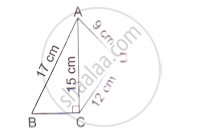# Find the Perimeter and Area of the Quadrilateral Abcd in Which Ab = 17 Cm, Ad = 9 Cm, Cd = 12 Cm, ∠Acb=90° and Ac = 15 Cm. - Mathematics

Find the perimeter and area of the quadrilateral ABCD in which AB = 17 cm, AD = 9 cm, CD = 12 cm, ∠ACB=90° and AC = 15 cm.#### Solution

In the right angled : ΔACB

AB^2=BC^2+AC^2

⇒ 17^2=BC^2+15^2

⇒ 17^2-15^2=BC ^2

⇒ 64=BC^2

⇒BC=8 cm

Perimeter=AB+BC+CD+AD

=17+8+12+9

= 46 cm^

Area of ΔABC=1/2(bxxh)

=1/2(8xx15)

=60 cm^2

AC^2=AD^2+CD^2

So, ΔADC is a right- angled triangle at D

Area of ΔADC=1/2xxbxxh

=1/2xx9xx12

=54 cm^2

∴ Area of the quadrilateral  = Area of ΔABC+Area of ΔADC

=60+54

=114 cm^2

Concept: Area of Circle
Is there an error in this question or solution?

#### APPEARS IN

RS Aggarwal Secondary School Class 10 Maths
Chapter 17 Perimeter and Areas of Plane Figures
Q 26